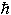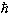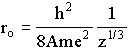Einstein's Theory of Relativity

## Classical Mechanics

by   Paul Marmet
( Last checked 2017/01/15 - The estate of Paul Marmet )
INTRODUCTION -
As seen in chapter one, the size of the hydrogen atom depends directly on the Bohr radius, which itself varies with the mass of the electron. Is that the case for all atoms? And what about molecules and crystals? Before we answer these questions rigorously, let us try to answer them intuitively.
Consider for example the hydrogen molecule, H2. It is made of two hydrogen atoms sharing their electrons. Since the size of the two hydrogen atoms taken separately varies with the Bohr radius, it would be reasonable to expect the size of the hydrogen molecule to do the same. If the radius of all atoms depended on the Bohr radius, we could apply the same reasoning to all molecules and crystals. Intuitively, we would arrive to the conclusion that the dimensions of matter depend on the Bohr radius. If this were to be the case, then according to chapter one, the size of any object would be different depending on its location in a gravitational potential. In this appendix, we will see how the dimensions of matter are predicted to vary theoretically. We will first look at all atoms. We will then study molecules which will be followed by crystals and metals.

Before we start our study of the dimensions of matter, a comment needs to be made about the Bohr radius and its use. Until now, ao has always been considered a constant because,eo, e and me have been supposed constants. With this in mind, most experimentalists present their results in units of bohrs using 1 bohr = ao = 5.29177×10-11 m  (page 349). For an experimentalist, by definition, that numerical value is equal to one bohr unit whether the electron orbit in hydrogen is constant or not.
For theoretical results, this is different. Theoreticians could decide to give the results of their calculations in function of ao (i.e. in units of ao) to be able to compare them to the experimentalists' results. For the theoreticians, ao is defined as a combination of parameters. Therefore ao is constant only if all the parameters are constant. One then has to be careful in reading theoretical results and look at the method used to see if there really is a dependence of ao or if it is just a unit. Let us make sure that the physics is not lost in those calculations.
Most authors do their calculations in atomic units. In those units, me = e == 1. This means that the unit of mass is the electron mass. When the Schrödinger equation (or the Dirac equation) is expressed in those units, we end up with an equation that seems independent of me. The authors then go on with numerical calculations to solve the equations. But if the mass of the electron is not a constant, then it is not necessarily equal to one in atomic units (with respect to the initial frame of reference). This changes the Schrödinger (or Dirac) equation which changes its solution which changes the value of the parameter we are looking for (e.g. the bond length or the radius of an atom in the initial frame of reference). All the results in this appendix being theoretical, we made sure that their dependence in ao was real.
ATOMS -
It is easy to derive the radius of all hydrogenlike atoms by supposing that they are just like a hydrogen atom with an electron orbiting a nucleus of charge Z. According to Levine  (page 525):

"The average radius of a hydrogenlike atom is proportional to the Bohr radius ao, and ao is inversely proportional to the electron mass".
The radius of all other atoms has been well investigated [2, 3] and the results given are proportional to the Bohr radius. The method used in  was the Hartree-Fock method  and in , the Dirac-Fock method which is just the Hartree-Fock method with relativistic corrections due to the mass of the electron with respect to the nucleus frame of reference. The Dirac-Fock method gives no relativistic correction of the electron mass with respect to an external gravitational potential.

THE HYDROGEN MOLECULE ION -
The hydrogen molecule is composed of two hydrogen atoms, each made of one electron and one proton. Its positive ion, H2+ , made of two protons and one electron, is a system that can easily be solved [1, 5, 6]. Upon finding its wave function and the potential of the nucleus (in the Born-Oppenheimer approximation), it is possible to calculate the distance between the two protons. This gives 2.00ao. (The variational method is used to solve this problem . It uses wave functions of the hydrogen atom which depend on the Bohr radius.) The internuclear distance of a molecule is in direct relationship with the size of that molecule. We see then that the size of the hydrogen molecule ion is proportional to ao .
This means that when we change the mass of the particle moving about the nucleus, the size of the hydrogen molecule ion also changes. This has already been realized by Levine  (page 355):

"The negative muon (symbolm-) is a short-lived (half-life 2×10-6 s) elementary particle whose charge is the same as that of an electron but whose mass mmis 207 times me. When a beam of negative muons (produced when ions accelerated to high speed collide with ordinary matter) enters H2 gas, a series of processes leads to the formation of muomolecular ions that consist of two protons and one muon. This species, symbolized by (pmp)+, is an H2+ ion in which the electron has been replaced by a muon. Its Re [the distance between the two protons] is 2.002/(mme2) = 2.002/(207mee2) = (2.00/207) bohr = 0.0051Å."

It is about one hundred times smaller than the Bohr radius. If one day we are able to produce a molecule with a proton and an anti-proton, the internucleus distance of that molecule will be amazingly small. It is obvious from this result that the size of the hydrogen molecule ion depends on the electron mass.

OTHER MOLECULES -
A lot of calculations have been done to find the size of molecules (i.e. the length of the bonds in the molecule) [7, 8, 9]. Some of the molecules studied include F2, Cl2, LiCl, Ni , HF and HCl. For heavier molecules, the calculations were done using internal relativistic corrections [10, 11, 12] because of the higher mass of the electron. Relativistic corrections due to an external gravitational potential were never taken into account. Some of the molecules studied in this way are N2, N2+ , Au2, AuH, AuCl, Cl2, F2, Xe2, Xe2+ , TlH and Bi2. The table published by Pyykkö  is extensive and covers more than one hundred molecules. All the results cited in the references are in units of ao or in units that are related to ao and are proportional to ao.

CRYSTALS AND METALS -
According to Zhdanov  (page 201), the equilibrium distance between particles in a crystal is proportional to the equilibrium spacing in a diatomic molecule having the same parameters for the potential energy. (The constant of proportionality depends only on the structure of the crystal.) This means that the size of crystals is proportional to the Bohr radius since we have seen in the previous section that the size of all molecules (and thus the distance between the nuclei in diatomic molecules) is proportional to the Bohr radius. Furthermore, the same author  (pages 208-209) develops an ionic model for metals. According to this model, the atomic radius in a metallic crystal (which is defined as half the shortest interatomic distance) can be expressed as:where h is Planck's constant, A is Madelung's constant, m is the electron mass, e is the charge of the electron and z is the valency of the atom. We see then that the size of metals is proportional to the Bohr radius as defined in chapter one.

CONCLUSION -
It is obvious that the size of all matter is strongly dependent on the Bohr radius and therefore the mass of the electron. Even if relativistic corrections are applied internally using Dirac's calculations, this correction does not take into account the relativistic effect caused by an external gravitational potential. This means that, since every object we know is made of either atoms, molecules, crystals or metals, the results of chapter one concerning the dilation and contraction of the Bohr radius in the hydrogen atom apply to all matter including humans. Finally, we conclude that this dilation or contraction is real.

REFERENCES
 Levine, Ira N., Quantum Chemistry, Prentice Hall, Englewood Cliffs, New Jersey, 1991, 629 pages.
 Froese Fischer, Charlotte, Average-Energy-of-Configuration Hartree-Fock Results for the Atoms Helium to Radon, Atomic Data and Nuclear Data Tables, volume 12, page 87, 1973.
 Desclaux, J. P., Relativistic Dirac-Fock Expectation Values for Atoms with Z=1 to Z=120, Atomic Data and Nuclear Data Tables, volume 12, page 311, 1973.
 Froese, Charlotte, Numerical Solution of the Hartree-Fock Equations, Canadian Journal of Physics, volume 41, page 1895, 1963.
 Cohen-Tannoudji, Claude, Bernard Diu et Franck Laloë, Mécanique quantique, Hermann, Paris, 1986, 1518 pages.
 McWeeny, Roy, Coulson's Valence, Oxford University Press, Oxford, 1979, 435 pages.
 Christiansen, Phillip A., Yoon S. Lee and Kenneth S. Pitzer, Improved Ab Initio Effective Core Potentials for Molecular Calculations, Journal of Chemical Physics, volume 71, number 11, page 4445, 1979.
 Noell, J. Oakey, Marshall D. Newton, P. Jeffrey Hay, Richard L. Martin et Frank W. Bobrowicz, An Ab Initio Study of the Bonding in Diatomic Nickel, Journal of Chemical Physics, volume 73, number 5, page 2360, 1980.
 Hay, P. Jeffrey, Willard R. Wadt et Luis R. Kahn, Ab Initio Effective Core Potentials for Molecular Calculations. II. All-Electron Comparisons and Modifications of the Procedure, Journal of Chemical Physics, volume 68, number 7, page 3059, 1978.
 Pyykkö, Pekka, Relativistic Effects in Structural Chemistry, Chemical Reviews, volume 88, page 563, 1988.
 Ziegler, Tom, Calculation of Bonding Energies by the Hartree-Fock Slater Transition State Method, Including Relativistic Effects, in Relativistic Effects in Atoms, Molecules and Solids, G. L. Malli editor, Plenum Press, New York, page 421, 1981.
 Ermler, Walter C., Richard B. Ross et Phillip A. Christiansen, Spin-Orbit Coupling and Other Relativistic Effects in Atoms and Molecules, Advances in Quantum Chemistry, volume 19, page 139, 1988.
 Zhdanov, G. S., Crystal Physics, Academic Press, London, 1965, 500 pages.

=========================

Chapter12 Contents    Appendix 2